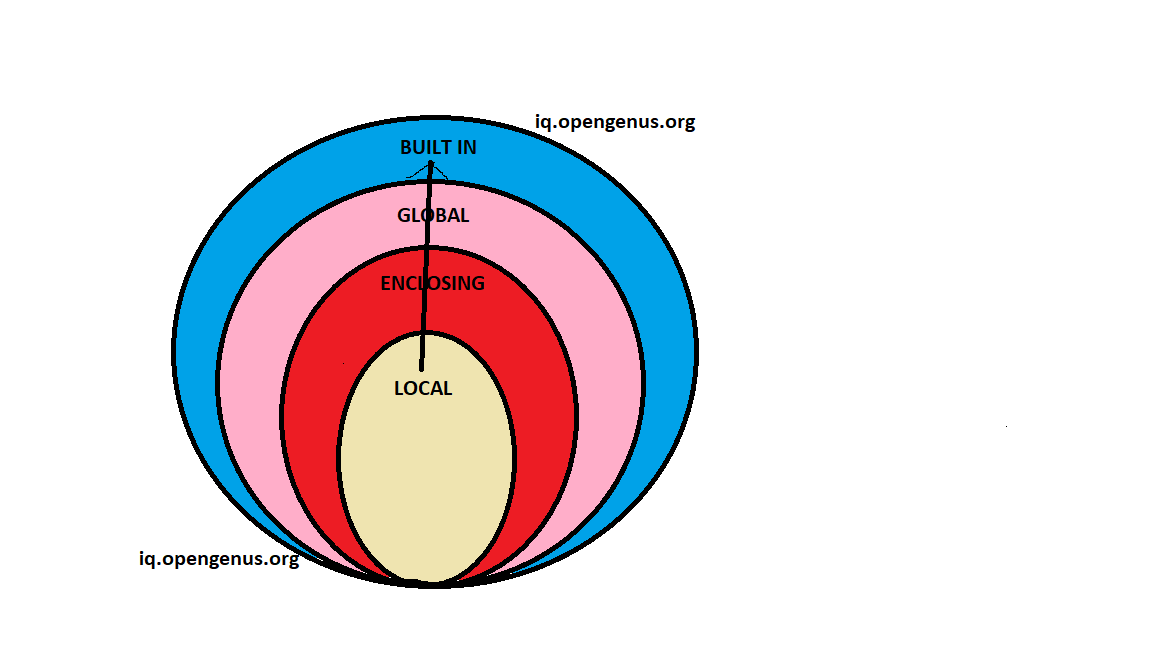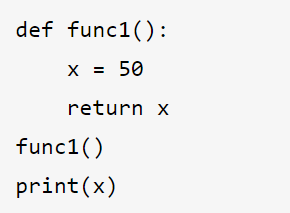×

Search anything:

# Function scope in Python

#### Software Engineering PythonGet this book -> Problems on Array: For Interviews and Competitive Programming

Hey programmer in this article we are going to explore about function scope in Python. Function scope in Python means how a particular function is accessible from different components depending on LEGB (Local -> Enclosing -> Global -> Built-in) rule.

Let's begin by recalling what function are.

## What is function in programming?

A function is a block of code containing related statements that perform specific tasks and it helps to break our program into smaller and modular chunks.

#### DON'T REPEAT YOURSELF

Fun fact - Block of code of a function runs only when function is called.
let's consider a simple example:

``````def my_function():
print("Hello")
``````

Now we have the basic idea about the function and let's recall about scope.

#### What does scope refers to?

Scope means Extent or range of view.But a programmer would think scope as the availability of a particular thing from inside the region it is created.

LEGB Rule

LEGB (Local -> Enclosing -> Global -> Built-in) is the logic followed by a Python interpreter when it is executing your program.Local Scope
Whenever a variable is defined within a function, its scope lies ONLY within the function.It is accessible from the point at which it is defined until the end of the function.

Fun fact- The local variable defined in the function exists for as long as the function is executing.Its value cannot be changed or even accessed from outside the function.

``````def print_num():
num = 1
# printing local variable num
print("The number defined is: ",num)

print_num()
# tring to print the value of num outside of function
print("The first number defined is: ",num)
``````

NOTE*- When we try to print the value of the local variable of the fucntion we get "NameError: name 'num' is not defined"

Enclosing Scope or Non-Locan scope
Enclosing scope is the scope of the outer or enclosing function.This is the case when we define function defined inside another function. Let's see how does the scope change by considering an example:

``````def outer():
num1 = 1
def inner():
num2 = 2
# Scope: Inner
print("In the function inner")
print("first num from outer: ", num1)
# Scope: Inner
print("second num from inner: ",num2)
inner()
# Scope: Outer
print("In the function outer")
print("second num from inner: ",num2)

outer()
``````

NOTE- When we try to print num2 outside the scope where it is defined we get an error "NameError: name 'second num' is not defined"

Nonlocal Keyword
This keyword basically provides more flexibility with variable scopes.The nonlocal keyword is useful in nested functions. It causes the variable to refer to the previously bound variable in the closest enclosing scope.

Syntax - nonlocal variable_name

``````def outer():
num1 = 1
def inner():
nonlocal num1
num1 = 0
num2 = 1
# Scope: Inner
print("In the function inner")
print("inner - num2 is: ",num2)
# Scope: Outer
inner()
print("In the function outer")
print("outer - num1 is: ", num1)

outer()

``````

NOTE-When we run this we get the value of num2=1 and num1=0.Let us have some food for brain.Notice what does nonlocal keyword just did

Global Scope
Whenever a variable is declared and defined outside any function, it becomes a global variable, and its scope is anywhere within the program. Which means it can be used and modified by any function.

``````def f():
s = "is fun."
print(s)

# Global scope
s = "Writing article in OpenGenus"
f()
print(s)
``````

Global Keyword
When we use global keyword in the function we are telling Python to use the globally defined variable instead of locally creating one.

Syntax - global variable_name

``````msg1 = "Welcome"

def change_msg(new_msg):
global msg1
msg1 = new_msg

def print_msg():
msg2 = "to my article"
print(msg1, msg2)

change_msg("Hi Welcome")
print_msg()

``````

Built-in Scope
Built in scope consists of all the reserved keywords.In simple words, Keywords are special reserved words. They are kept for specific purposes and cannot be used for any other purpose in the program.When used inappropriately they raise an error.
It's important to note that we can use them anywhere in the function and we don't need to declare or define it before using them.

• and -- A logical operator
• as -- To create an alias
• assert -- For debugging
• break -- To break out of a loop
• class -- To define a class
• continue -- To continue to the next iteration of a loop
• def -- To define a function
• del -- To delete an object
• elif -- Used in conditional statements, same as else if
• else -- Used in conditional statements
• except --Used with exceptions, what to do when an exception occurs
• False-- Boolean value, result of comparison operations
• finally -- Used with exceptions, a block of code that will be executed no matter if there is an exception or not
• for --To create a for loop
• from--To import specific parts of a module
• global-- To declare a global variable
• if--To make a conditional statement
• import-- To import a module
• in To check if a value is present in a list, tuple, etc.
• is-- To test if two variables are equal
• lambda -- To create an anonymous function
• None -- Represents a null value
• nonlocal-- To declare a non-local variable
• not-- A logical operator
• or-- A logical operator
• pass-- A null statement, a statement that will do nothing
• raise-- To raise an exception
• return-- To exit a function and return a value
• True-- Boolean value, result of comparison operations
• try-- To make a try...except statement
• while-- To create a while loop
• with-- Used to simplify exception handling
• yield --To end a function, returns a generator

Let's recall what we have learnt by taking a small quiz.## Question

#### What is the output of the above code?

50
NameError
Runtime Error
Compiler Error

Python Scope vs Namespace
In Python, the concept of scope is closely related to the concept of the namespace. As you’ve learned so far, a Python scope determines where in your program a name is visible. Python scopes are implemented as dictionaries that map names to objects. These dictionaries are commonly called namespaces.

Referre
Hope this helped you.

Cheers to all the Python programmers around the globe!!

Have a good day :)